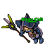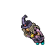## Wednesday, March 3, 2021

### TibiaGoals Riddles E6R6

Comment down below!

1.3 x 2 = 6

Shizurie

2.3.take top left arrow of the '9' to make it a '3' and move it to the bottom left of '5' to makte into a '6'. 3x2=6

Impure

4.Transform the 9 into a 3, and the 3 into a 6
3 x 2 = 6

Char: Marulina

5.6.Heya! :D
Take the upper-left arrow from the 9 and put it to the lower-left of the 5; the equation now becomes 3x2=6. :)
Thanks and have a great day! :D

7.3 × 2 = 6
Mian Stone'arrow

8.3x2=6

Lovely Olka

9.10.11.12.13.Aribebech
3x2=6

14.The arrow top west of 9 /move it to the bottom west of 5
Then the equation will be 3x2=6

Skyelie<3

15.16.From the number 9 we remove an arrow, from 9 it goes to the number 3, that arrow we add to the number 5, to turn it into a number 6. The equation would be like this: 3 x 2 = 6

17.From the number 9 we remove an arrow, from 9 it goes to the number 3, that arrow we add to the number 5, to turn it into a number 6. The equation would be like this: 3 x 2 = 6

18.19.3x2=6

Eternal Hunger
Antica

20.3x2=6
By moving vertical arrow from 9 to 5

Eternal Hunger
Antica

21.3x2=6
By moving vertical arrow from 9 to 5

Eternal Hunger
Antica

22.By moving the upper left vertical arrow of the number 9 to the bottom left of number 5 so they form a 3 and a 6 instead. 3 x 2 = 6.

-Essiee

23.Respuesta 3*2=6 .... Bebitha consentida

24.9 x 2 = 5 la respuesta corresta es el 9 lo colocamos 3 y el 5 se coloca 6 seria la respuesta : 3 x 2 = 6 .... Zazy torturador sombrio

25.Nehkz
Relania

3x2=6

26.Nehkz
Relania

3x2=6

27.Nehkz
Relania

3x2=6

28.3x2=6 Raul Charlover

29.The equation can be corrected by removing one arrow from the 9 to create a 3.
Then placing the arrow on the 5 to create a 6.
3 x 2 = 6

Character: Elee Bae

30.Turning the 9 into 3 & turn the 5 into 6
3 x 2 = 6

Char: Dyaniixz

31.Moonizera: 3x2=6

32.R= 3 x 2 = 6
Samuray de carro

33.cambiando la flecha de arriba izquierda del 9 para hacerlo un 3 y usar esa flecha para convertir el 5 en 6

34.3 x 2 = 6
Character: Amy Meow

35.3x2 = 6 (Moving one arrow from 9 to 5, making 9 a 3, and 5 a 6)
Dorrohov

36.change the number 9 to 3 and using the arrow the make the 5 to 6
Knight Narco

37.3x2 = 6
Sir wuachachucho

38.3x2=6
Vysterion

39.Zuali

https://gyazo.com/6c1038e8ecf45e814e1bf47ca6cebbdf

40.3x2=6 - Mikolaj

41.3x2=6 - Mikolaj

42.3x2=6 - Mikolaj

43.3 x 2 = 6

PAMELIIN

44.Myo Disturbed

By moving the upper left arrow from the nine to the five and make it 3 x 2 = 6

45.Top left arrow of the 9 you can move that vertical arrow from the 9 (making it a 3), over to the bottom left of the 5, making it a 6. So the equation would be 3x2=6 making it correct.

46.47.Congratulations to all of you! You did great, as always!

Sadly, some of you won't qualify to this event lottery anymore. Those are:
- Norelli (3rd, 4th, 6th riddle missing)
- Procente (2nd, 3rd, 6th riddle missing)

48.1.